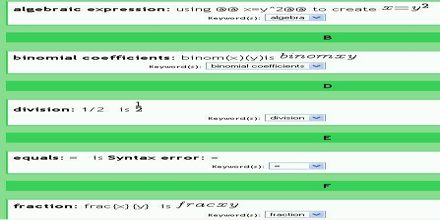Mathematic

# Introductory Algebra GlossaryThe aim of this lecture is to present on Introductory Algebra Glossary. Here also focus on quadratic equation. A quadratic equation is anequation that can be written in the form ax2 + bx + c = 0, where a, b, and c are real numbers,with a 0. This lecture also focus on; complex number: any number that can be written in the form a + bi, where a and b are real numbers; and Parabola: The graph of a second-degree (quadratic) equation in two variables is called a parabola.